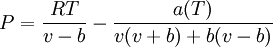# Difference between revisions of "Peng and Robinson equation of state"$P = \frac{RT}{v-b} - \frac{a(T)}{v(v+b) +b(v-b)}$